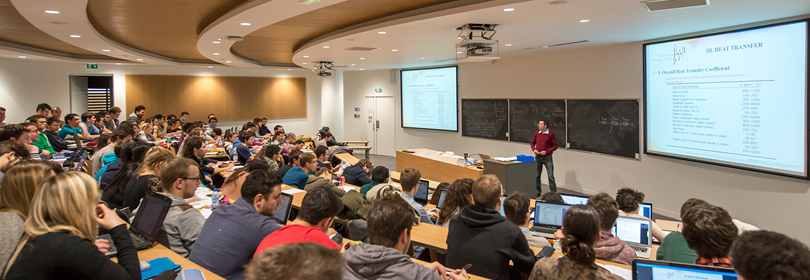Our engineering & master degrees
Ecole nationale supérieure de l'Énergie, l'Eau et l'Environnement
-
Engineering school in energy, water and environment# - 3EUS2MKS

A+Augmenter la taille du texteA-Réduire la taille du texteImprimer le documentEnvoyer cette page par mail cet article Facebook Twitter Linked In
• #### Number of hours

• Lectures : 14.0
• Tutorials : 14.0
• Laboratory works : 22.0
• Projects : 0
• Internship : 0
ECTS : 2.5

### Goals

Provide basic notions required for the mechanical design of rotating machines for energy production.

1- Introduction to the theory of vibrations:
Introduce Lagrange equations and solve the equations of motion in simple cases and for small oscillations around equilibrium

2- Linear elasticity of solid bodies:
Understand concepts of stress and strain
Introduce linear elastic consitutive law
Understand the concept of elastic criterion
Structural design using a finite-element method program

Contact Barthelemy HARTHONG

Content

1- Introduction to the theory of vibrations:
1- One efficient method for solving the equations of motion
Description of the motion of a mechanical system using generalised coordinates
Principle of virtual power and Lagrange equations
Linearisation of the equations of motion
Free and forced oscillations of a one-degree-of-freedom system
Eigenfrequencies, eigenmodes
Vibration of systems with several degrees of freedom
2- numerical intégration of the equations of motion

2- Linear elasticity of solid bodies:
1- Basics of the theory of elasticity
Stress
Strain
linear elastic constitutive law
Mises limit criterion
Introduction to a finite-element program, mesh, boundary conditions
Comparison with analyticam solutions of simple problems, torsion, bending, traction, shear
Principal stresses and strains
Design exercices - Mises limit criterion

Prerequisites

Basics of mathematics - volume and surface integrals, differential equations, Taylor series, vector analysis, linear algebra

Tests

2h exam + assignment

2/3 DS + 1/3 CC

Curriculum->->Semester 2

Bibliography

Seto, Mechanical Vibrations, Schaum's outline series, McGraw Hill, 1964
Landau, Lifchitz, Mécanique, Editions Mir 1966
Lalanne, Berthier, Der Hagopian, Mécanique des vibrations linéaires, Masson, 1986

A+Augmenter la taille du texteA-Réduire la taille du texteImprimer le documentEnvoyer cette page par mail cet article Facebook Twitter Linked In

Date of update February 8, 2017